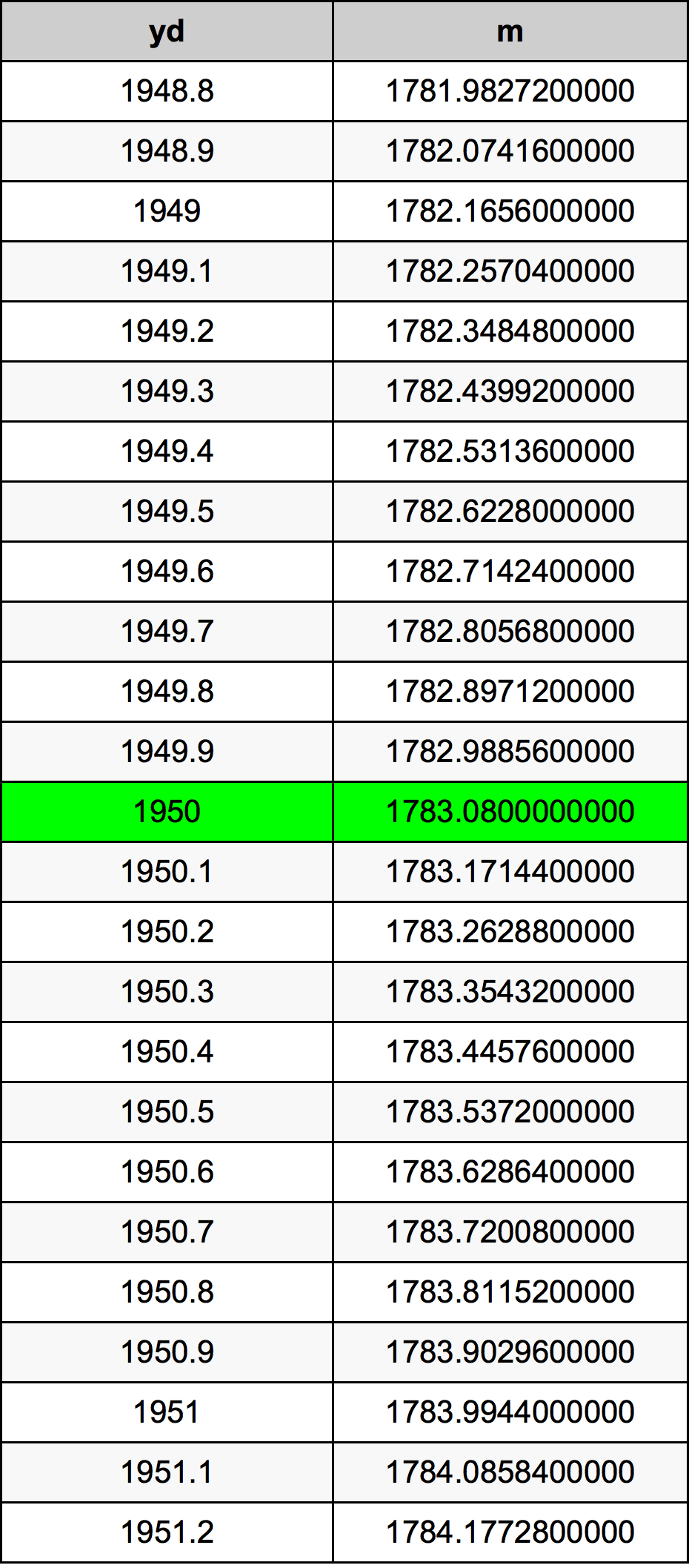Yards To Meters

# 1950 yd to m1950 Yards to Meters

yd
=
m

## How to convert 1950 yards to meters?

 1950 yd * 0.9144 m = 1783.08 m 1 yd
A common question is How many yard in 1950 meter? And the answer is 2132.54593176 yd in 1950 m. Likewise the question how many meter in 1950 yard has the answer of 1783.08 m in 1950 yd.

## How much are 1950 yards in meters?

1950 yards equal 1783.08 meters (1950yd = 1783.08m). Converting 1950 yd to m is easy. Simply use our calculator above, or apply the formula to change the length 1950 yd to m.

## Convert 1950 yd to common lengths

UnitLengths
Nanometer1.78308e+12 nm
Micrometer1783080000.0 µm
Millimeter1783080.0 mm
Centimeter178308.0 cm
Inch70200.0 in
Foot5850.0 ft
Yard1950.0 yd
Meter1783.08 m
Kilometer1.78308 km
Mile1.1079545455 mi
Nautical mile0.9627861771 nmi

## What is 1950 yards in m?

To convert 1950 yd to m multiply the length in yards by 0.9144. The 1950 yd in m formula is [m] = 1950 * 0.9144. Thus, for 1950 yards in meter we get 1783.08 m.

## 1950 Yard Conversion Table## Alternative spelling

1950 Yards to m, 1950 Yards in m, 1950 yd to Meter, 1950 yd in Meter, 1950 Yards to Meters, 1950 Yards in Meters, 1950 yd to Meters, 1950 yd in Meters, 1950 Yards to Meter, 1950 Yards in Meter, 1950 Yard to m, 1950 Yard in m, 1950 Yard to Meter, 1950 Yard in Meter